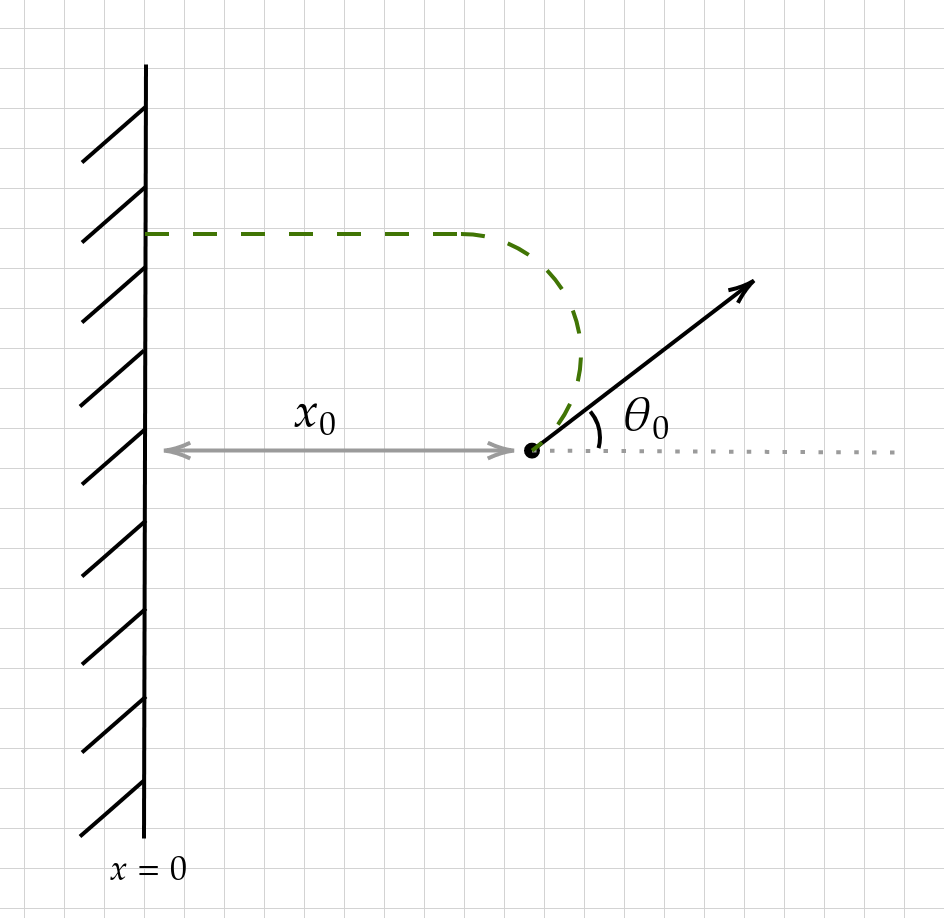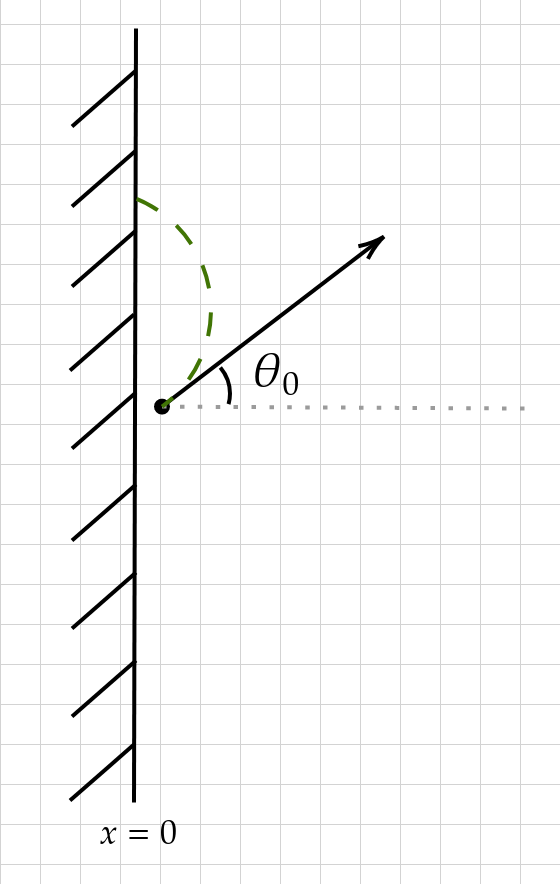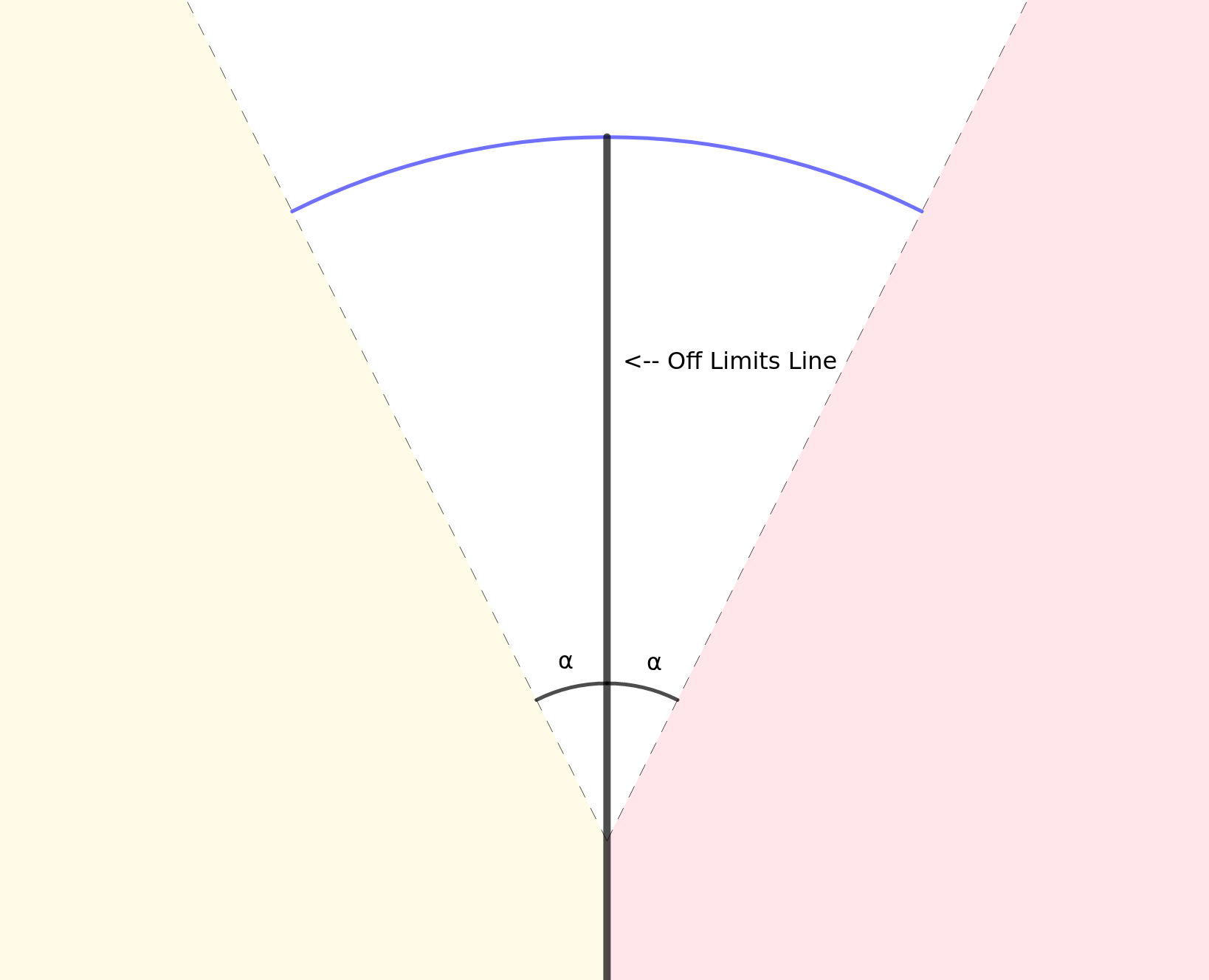July 2022 > Math

# Easy Questions about Dubins Paths

This post is about Dubins path planning; see the previous post here (Dubins Corner Revisited) for context and application for the following problems. With the resolution of the following lemmas, the proof of the Dubins' corner problem in the previous post will be compete.

The term "Easy" should not be taken in the pejorative sense. Instead, they are path planning problems with simple statements, simple answers, and simple proofs. In fact, the shortest path in each case will be so blatantly obvious that it almost does not need a proof. On the other hand, I have failed to supply the kind of one-line proof that my expectation of elegance demands, which makes these problems excellent candidates for the sort of informal minimal-proof competitions that math students tend to enjoy. I also have a lingering interest in keeping the following arguments as elementary as possible. I have managed to avoid using any result specific to Dubins path planning at all, notably the characterization of shortest Dubins paths on a plane. This further signifies that the Dubins Corner problem is in fact very "easy".

## Question #1: Shortest Path to a Line

(Shortest Path to a Line) Let $$(x_0,y_0)\in \mathbb{R}^2$$ be the initial position of a Dubins car, $$x_0>0$$, and $$\theta_0\in [0, 2\pi)$$ be the initial orientation of the car, measured as an angle from the positive $$x$$-axis. The shortest path from $$(x_0,y_0,\theta_0)$$ to any point on the $$y$$-axis is formed by an arc of radius 1, possibly followed by a line parallel to the $$x$$-axis. If $$\theta_0\in (0,\pi)$$, the arc is a left turn, if $$\theta_0\in (\pi, 2\pi)$$ the arc is a right turn. If $$\theta=0$$, both a left and right turn yield shortest paths (the unique case of multiple solutions), otherwise if $$\theta=\pi$$ the arc has zero length. The car follows the arc until it either hits the $$y$$-axis or becomes parallel to the $$x$$-axis, whichever comes first. If it has not hit the $$y$$-axis, then it continues straight until it does.The images above illustrate the solution. The shortest path is essentially a greedy path on $$\theta(t)$$, achieving and maintaining $$\theta(t)=\pi$$ as quickly as possible. This will be the substance of our proof below.

Proof. Let $$\theta(t)$$ be the angle of the Dubins car at a time $$t$$. We seek an assignment of $$\theta(t)$$ so that $x(t)=x_0+\int_0^t \cos(\theta(s))\,ds$ is zero as quickly as possible. Let $$\widehat{\theta}(t)$$ be the angle of the proposed optimal path, and $$\theta(t)$$ be another Dubins path. Since $$|\widehat{\theta}'(t)| \leq 1$$ (radius restriction), the function $$|\pi-\widehat{\theta}(t)|$$ is pointwise less than or equal to $$|\pi - \theta(t)|$$. Thus \begin{align*} \cos(\widehat{\theta}(t))&=-\cos(\pi-\widehat{\theta}(t))=-\cos(|\pi-\widehat{\theta}(t)|) \\ &\leq -\cos(|\pi-\theta(t)|)=-\cos(\pi-\theta(t))=\cos(\theta(t)) \end{align*} and $\int_0^t \cos(\widehat{\theta}(s))\,ds \leq \int_0^t \cos(\theta(s))\,ds\,.$ This shows that $$\widehat{\theta}$$ is optimal. If $$|\pi-\theta(t)|$$ is strictly greater than $$|\pi-\widehat{\theta}(t)|$$ for some $$t$$, then there is some $$t$$ for which $$\cos(\widehat{\theta}(t))$$ is greater than $$\cos(\theta(t))$$ so that, by continuity, $\int_0^t \cos(\widehat{\theta}(s))\,ds \lneq \int_0^t \cos(\theta(s))\,ds\,.$ If $$\theta_0\neq 0$$, then $$|\pi-\theta(t)|=|\pi-\widehat{\theta}(t)|$$ implies that $$\theta(t)=\widehat{\theta}(t)$$ by continuity. Considering $$\theta=0$$ easily shows the two optimal paths which are mirror images of each other. (QED)

## Question #2: Shortest Path Through a Sector(Shortest Path Through a Sector) Consider two regions $$A=\{x>0, x>\tan(\alpha)y\}$$ and $$B=\{x<0, x<-\tan(\alpha)y\}$$ on the plane. The shortest Dubins path avoiding the line $$\{x=0,y<1\}$$ is a circular arc passing through the point $$(0,1)$$.

This situation is illustrated in the above picture. As is standard (and without loss of generality), we assume a minimum turning radius of 1.

Proof. Any Dubins path $$p$$ from $$A$$ to $$B$$ must pass through the line $$L=\{x=0,y\geq 1\}$$. We will show that the